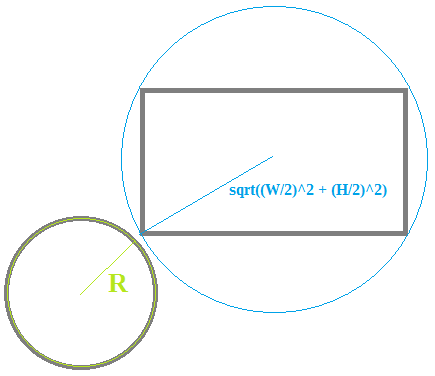# Game doesn't run smoothly

Hello people,

I am creating a game based off brick breaker, I set up the ball and paddle, and went to test it.
The game works fine, except for one issue, it seems that the game repeatedly stops and starts, kind of like a hiccup.

Does anyone know any way to fix this? Thanks.

How many collision tests are you doing per frame (how many bricks there are)?

Try this, instead checking collision with every brick, check the collision with close bricks only, this may improve the performance or may not:

```Conditions: Squared distance between Ball and Brik is <= 200: Actions: No actions // Sub-event Conditions: Brick collides with Ball Actions: Bounce Ball Delete Brick```

To check the squared distance (squared, not sqrt(), to make it faster) you have to use the “Compare two expressions” condition:
*In the first parameter: Ball.SqDistance(Brick), this is the squared distance.
*The third parameter would be the squared of the sum of the ball radius and brick’s bound circle radius, it’s a constant, don’t calculate it in GD every frame, calculate it only one time or calculate it yourself and put the result:As you can see, the Ball radius is = Ball.Width() = Ball.Height(), and Brick bound radius is = sqrt(pow(Brick.Width()/2, 2) + pow(Brick.Height()/2, 2)).
Ball radius + Brick bound radius is the min distance to collide, to get the squared distance, do (Ball radius + Brick bound radius)^2
In GD it would look this way:

As I said, It may work or may not